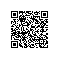### 特征描述子(Feature Descriptor)

（HOG特征描述子可以不局限于一个长度，也可以用很多其他的长度，这里只记录一种计算方法。）

### 怎么计算方向梯度直方图呢？

#### 第一步：预处理

Patch可以是任意的尺寸，但是有一个固定的比例，比如当patch长宽比1:2，那patch大小可以是100*200, 128*256或者1000*2000但不可以是101*205。hog_preprocess

#### 第二步：计算梯度图像// C++ gradient calculation.
img.convertTo(img, CV_32F, 1/255.0);

Mat gx, gy;
Sobel(img, gx, CV_32F, 1, 0, 1);
Sobel(img, gy, CV_32F, 0, 1, 1);


# Python gradient calculation

im = np.float32(im) / 255.0

gx = cv2.Sobel(img, cv2.CV_32F, 1, 0, ksize=1)
gy = cv2.Sobel(img, cv2.CV_32F, 0, 1, ksize=1)// C++ Calculate gradient magnitude and direction (in degrees)
Mat mag, angle;
cartToPolar(gx, gy, mag, angle, 1);

# Python Calculate gradient magnitude and direction ( in degrees )
mag, angle = cv2.cartToPolar(gx, gy, angleInDegrees=True)#### 第三步：在8*8的网格中计算梯度直方图8*8网格直方图

v = [128, 64, 32]
[(128^2) + (64^2) + (32^2) ]^0.5=146.64hog-16x16-block-normalization

### 第五步：计算HOG特征向量

1. 我们有多少个16*16的块？水平7个，垂直15个，总共有7*15=105次移动。

2. 每个16*16的块代表了36*1的向量。所以把他们放在一起也就是36*105=3780维向量。

#### 可视化HOGvisualizing_histogram

====================================分割线================================